Examples

Chapter 13 Class 12 Probability (Term 2)
Serial order wise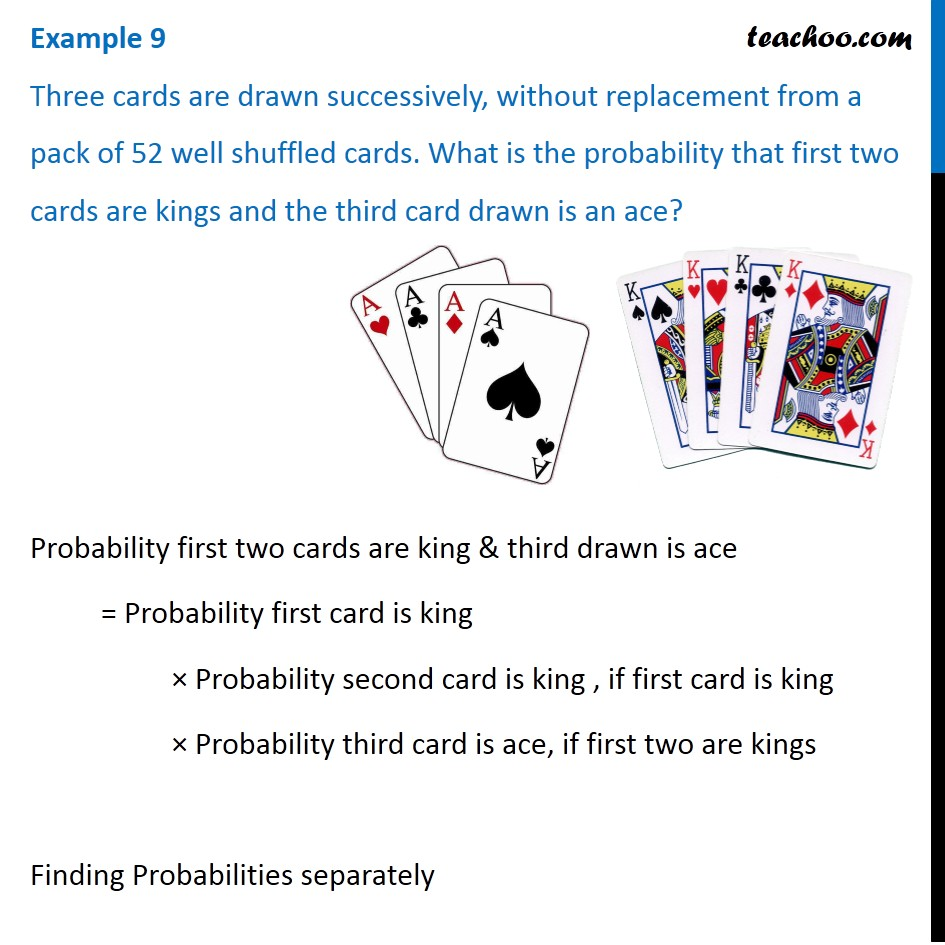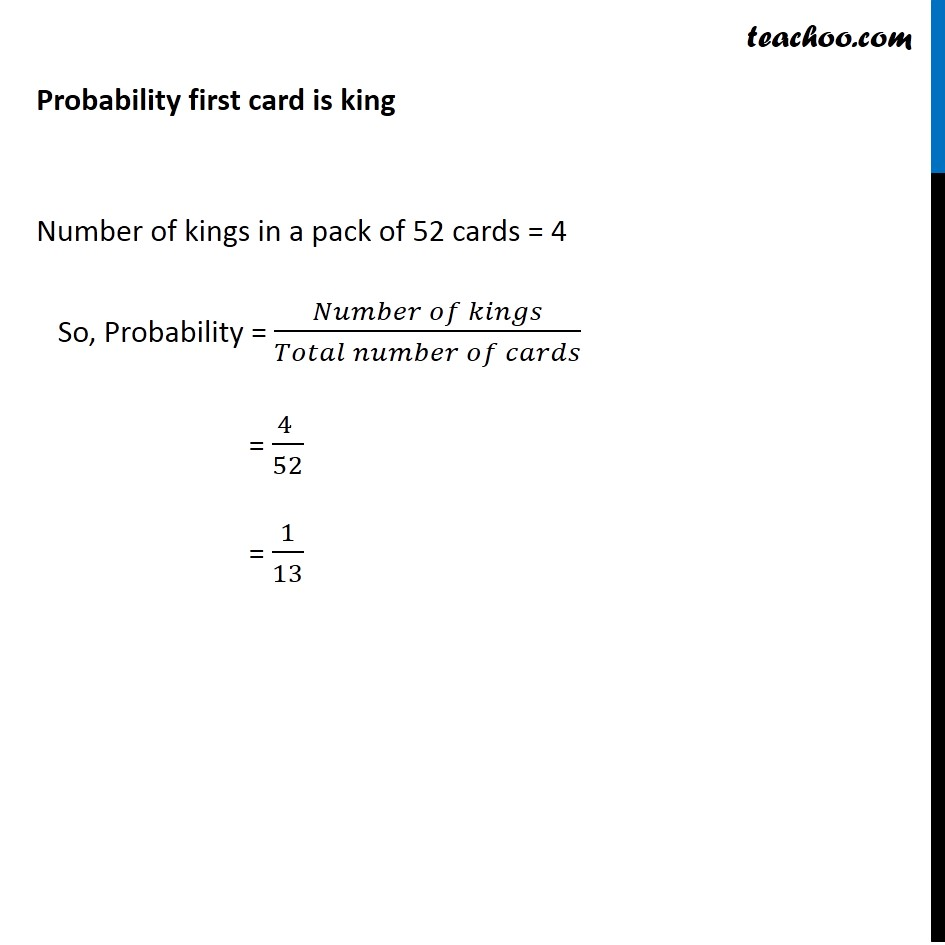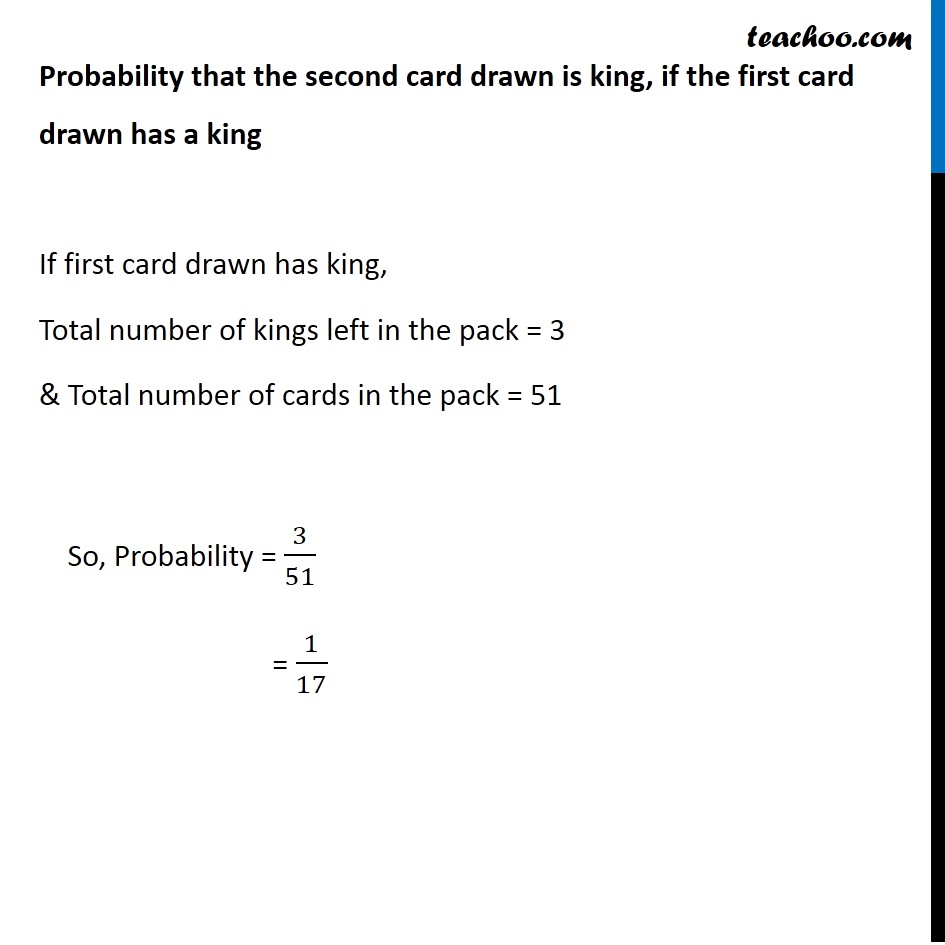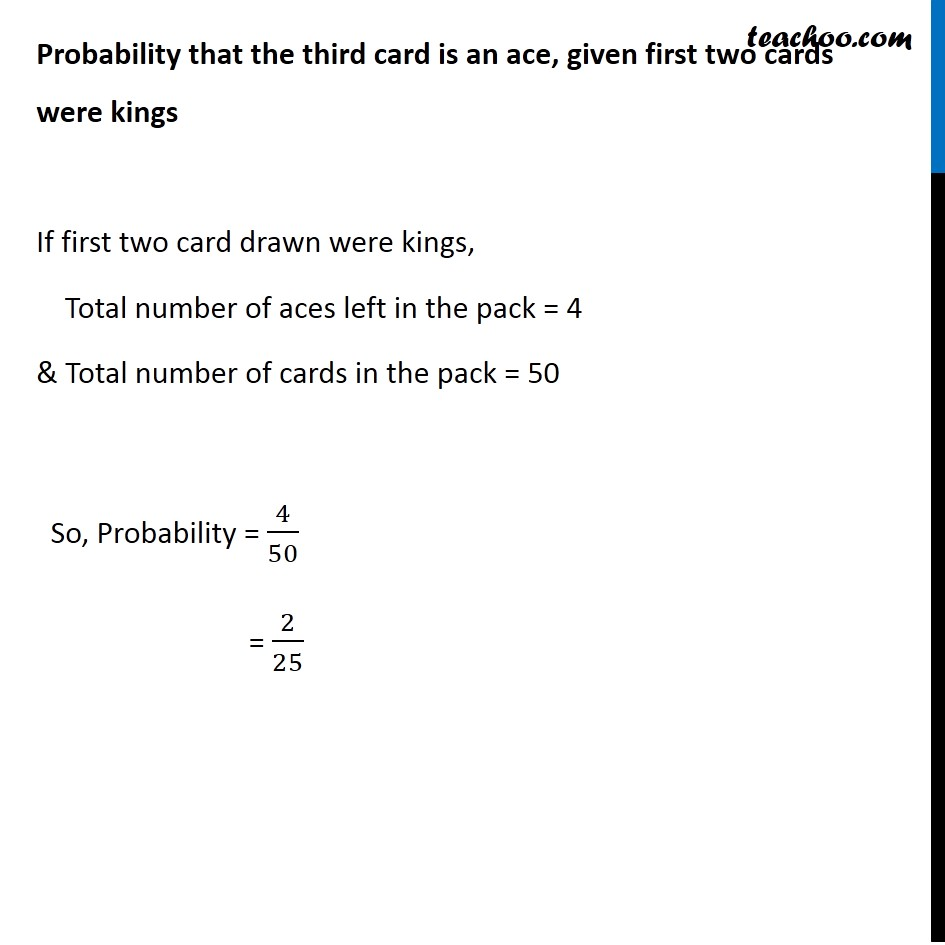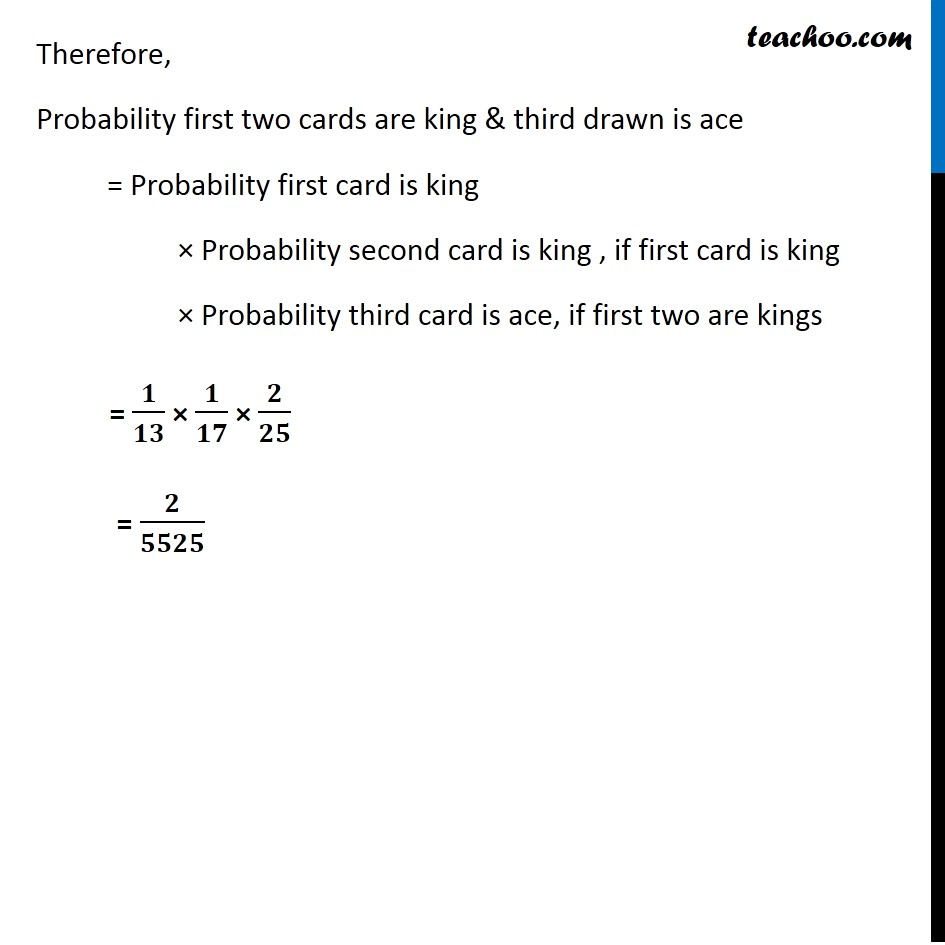### Transcript

Example 9 Three cards are drawn successively, without replacement from a pack of 52 well shuffled cards. What is the probability that first two cards are kings and the third card drawn is an ace? Probability first two cards are king & third drawn is ace = Probability first card is king × Probability second card is king , if first card is king × Probability third card is ace, if first two are kings Finding Probabilities separately Probability first card is king Number of kings in a pack of 52 cards = 4 So, Probability = (𝑁𝑢𝑚𝑏𝑒𝑟 𝑜𝑓 𝑘𝑖𝑛𝑔𝑠)/(𝑇𝑜𝑡𝑎𝑙 𝑛𝑢𝑚𝑏𝑒𝑟 𝑜𝑓 𝑐𝑎𝑟𝑑𝑠) = (4 )/52 = 1/13 Probability that the second card drawn is king, if the first card drawn has a king If first card drawn has king, Total number of kings left in the pack = 3 & Total number of cards in the pack = 51 So, Probability = 3/51 = 1/17 Probability that the third card is an ace, given first two cards were kings If first two card drawn were kings, Total number of aces left in the pack = 4 & Total number of cards in the pack = 50 So, Probability = 4/50 = 2/25 Therefore, Probability first two cards are king & third drawn is ace = Probability first card is king × Probability second card is king , if first card is king × Probability third card is ace, if first two are kings = 𝟏/𝟏𝟑 × 𝟏/𝟏𝟕 × 𝟐/𝟐𝟓 = 𝟐/𝟓𝟓𝟐𝟓